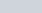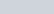If a quadratic equation has complex roots then they are complex conjugate pairs. In this video I show you how we can use this fact to find the quadratic equation knowing one of the roots.

#### Find the quadratic equation that has the following as one of its roots:

1.2.